Bell's Inequalities Revisited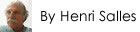Last Update March 26 2019

Abstract

Quantum mechanics statistics, no matter how spectacular, do not mandate uncertainty. Proof is a simple equation based on an hidden variable just as predicted by EPR.

1. Introduction

There are two schools of thoughts concerning entangled particles:

1. Along Bohr's thinking is Quantum Mechanics in which statistics and uncertainty rule. Uncertainty rules because entangled pairs are all identical to each other; no matter where or when emitted any entangled pair occurs under the same and unique quantum state made of eight simultaneous yet incompatible constitutions. When a particle of any pair is measured one of these eight outcomes haphazardly pops out; and the measured particle is instantaneously instructing over distance the companion particle to comply, a phenomenon known as non-locality; at that very time the pair's quantum state and its uncertainty vanish.
2. Along Einstein, Podolsky, Rosen or EPR paper  published in 1935 is conventional physics, or local realism, which asserts that behind quantum statistics and uncertainty are hidden variables; without questioning Quantum mathematics and statistical results EPR nevertheless challenges both uncertainty and nonlocality concepts attached to it.

Later in 1964, well after above controversy was mathematically confirmed and before any experiments were run, John Stewart Bell came up with his famous inequalities , establishing that should future physical experiments justify Quantum Mechanics, as summarized in section 1 above, there would be no hidden variables; that would contradict EPR and no Classical or local theory would ever be established.

In the 1980s physical experiments ran by Alain Aspect , and many others since, have definitely justified quantum statistics predictions.
Since then, in accordance with Bell's inequalities, the consensus is that there is no hidden variable and no local theory can be established; in essence declaring above EPR's conclusions wrong.

In 1980 N.D. Mermin wrote a paper  about Quantum Mechanics, which you might want to read; it explains in much more details and in simple terms Quantum Mechanics uncertainty and non-locality.

Challenging Bells' claim, and at same time justifying EPR prediction, this web page provides a local equation conforming to physical experiments just as Quantum Mechanics does; the equation provided describes the behavior of each and every individual particle of each and every individual entangled pair, a feature abolishing uncertainty.
And in the process Bell's inequalities are turned into equalities.

2. Conventions and the Mathematical Discord

2.1 Conventions

In order to describe the situation some conventions are needed. In the following the trigonometric notation is used; the 00 angular reference coincides to the entangled pairs trajectory also equal to 3600; and by definition positive angles are counted counter clockwise.
While entangled pairs are either two photons or two electrons particles in Quantum Mechanics, for simplicity this non-quantum or local interpretation deals with electrons only.2.2 Detectors orientations

Figure 1 represents Mermin's "Complete device" also as implemented in physical experiments. It is made of two detectors magnets A and B on either sides of an emitter C of entangled pairs of electrons. The detectors magnets are graphically represented by a circumference and a diameter line delineating the North Pole, labeled with a capital "N", from the South Pole "S" represented in grey. In Figure 1 detector A is oriented at 00 while detector B is oriented at 1200.

2.3 The two electron's magnetic orientations or tilts of any individual pair are identical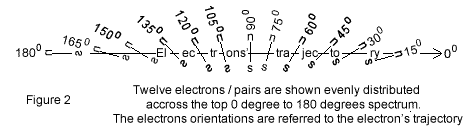An electron is made of a rotating electric charge acting as a magnet, which north pole is defined with the right hand rule; curving the right fingers along the electron's spin, the thumb points north, which defines the magnetic direction of the electron called tilt in this web page; here an electron is represented with a bar and two small "s" and "n" letters for their south and north poles.
And very specifically, according to Mermin's article [Page 942], the measures indicate that the two electrons of any pair are identical: "The detectors flash the same colors when they have the same settings because the particles carry the same instructions." This physical outcome, which is part of Quantum Mechanics, is also adopted as is in this non-quantum local physics; when measured both electrons magnetic orientations or tilts of an entangled pair are identical. As such a pair graphical representation, represents also both electrons tilts of the pair.
Note regarding the orientations of the 2 particles of an entangled pair. Overlap this line with your mouse pointer.
The particles orientations are angles counted positively counterclock wise with respect to their trajectory. The origin being at the Emitter C.
As such the observer on the right side of Figure 2 will measure a particle oriented at 60 degrees to have an orientation of 60 degrees. Yet the observer on the left of Figure 2 will see the entangled particle of the same pair oriented at 180 plus 60 degrees or 240 degrees.
This writer thinks that might be the reason for which entangled pairs are sometimes referred as being made of 2 complementary particles.
The interpretation of the measures is such that it ends up providing the right information whether the 2 particles are considered to be complementary or having identical orientations.
Figure 2 represents 12 pairs, which angular tilts are represented counter clock wise with respect to the electron's trajectory.
Figure 2 clearly emphasizes this local interpretation in which each electron's tilt is specifically determined.
In contradiction Quantum statistical mechanics asserts that the electrons have no magnetic orientation at all; all pairs share an identical and unique quantum state, which represents Quantum Mechanics uncertainty's very mathematical expression, and which defies human logic as detailed Figure 2.

2.4 Green versus Red measuresIn this local interpretation each electron magnetic orientation of each entangled pair is confronted to its detector and individually measured.
In Figure 3 the angles ΘA and ΘB (read theta) are the orientations respectively given to Detector A and Detector B magnets.
Figure 3 illustrates the measurement of a single entangled pair emitted with a 300 magnetic tilt; the 2 electrons are represented within their respective Detectors A and B.
If electron north pole matches detector North Pole, the detector flashes GREEN (or G) as Detector A does. When they do not match, they flash RED (R) as Detector B does.

2.5 Bell's theorem local interpretation

Before any experiments could be ran the following was asserted. Electron's tilted between 00 and 1200, with respect to their own motion orientation, represent two thirds of those tilted within the upper 00 to 1800 half circle; according to Figure 3 they will flash RED in detector B and GREEN in detector A; the remaining pairs tilted between 1200 and 1800, or one third of the upper half circle will flash GREEN in both detectors. The conditions below the electrons' trajectory from 1800 to 3600 being symmetrical to those above the electrons' trajectory, the same one third two thirds distribution applies.
And no matter the number of pairs considered, this reasoning applies.

When the detectors angles are ΘA = 00 and ΘB = 1200, as in Figure 3, this local interpretation provides one third same colors to two thirds different colors distribution.

2.6 Quantum Mechanics predictions

Mermin provides Quantum Mechanics equation, which was also established before any experiments took place: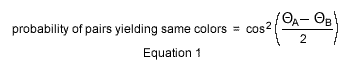The detectors orientations ΘA and ΘB can be each given any values from 00 to 3600. Quantum Mechanics Equation 1 provides the following same colors to different colors ratio.

When the detectors angles are ΘA = 00 and ΘB = 1200, as in Figure 3, this equation predicts that same colors (either GG or RR) will be flashed one-quarter of the times, and opposite colors (either RG or GR) will be flashed three-quarters of the time.

2.7 Science's stunning mathematical discord

The physical experiments and resulting measures, which occurred later, precisely matched Equation 1 results.
This situation, in which one third two thirds allegedly undeniable distribution contradicts the one quarter three quarters distribution predicted by Quantum Mechanics and finally found to comply with experiments, is stunning yet it remained undisputed until following proposed Equation 2.

3. Local Mathematics Conforming to Experiments

Provided the pairs are evenly distributed, those with tilts between 00 and 1350 represent three quarters of those above the electron's trajectory. The remaining pairs between 1350 and 1800 make the remaining quarter. And an identical distribution applies to the circle's lower half.
In order to match the physical measures and Quantum Mechanics statistics when the detectors are set 1200 apart, it suffices to come up with an equation that modifies the pairs tilted between 00 and 1350 labelled evenly distributed to angles tilted between 00 and 1200 now renamed reoriented. The pairs evenly distributed between 00 and 1800 are reoriented from Θelectron evenly distributed to Θelectron reoriented as follows: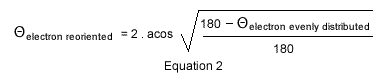When the detectors are oriented 1200 apart as in Figure 3, the electrons evenly tilted from 00 to 1350electron evenly distributed) when emitted will be reoriented and measured by Detector B between 00 and 1200electron reoriented) just as the measures show and just as predicted by Quantum Statistics.
Figure 4 illustrates Equation 2; Equation 2 predicts the behavior of each electron of each pair; while conforming to Quantum statistics, Equation 2 is dethroning the concept of uncertainty.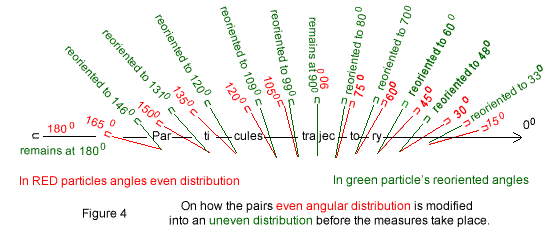And as already mentioned, because the 2 electrons of any pair when measured have identical deflection, each grey and black lines in Figure 4 represent a pair orientation as well as each electrons orientations of that pair.
In this interpretation the 2 electrons of any pair are acting in concert, not because one particle is instructing the other to comply over distance (Quantum Mechanics nonlocality interpretation), but because, before the measures take place, their individual yet common tilts have each been re-oriented in an equal amount as provided by Equation 2.
Please note that the 00, 1200 detectors setting as used in all of above, is not restrictive; the 2 detectors can be set at any of two values other than 00 and 1200; in all cases Equation 2 provides reoriented values that fit the experiments.
Equation 2 abolishes the former local one third two thirds distribution not conforming to reality in favor of one quarter three quarters distribution conforming to reality.
Equation 2 local reorientation implies the following:
• To start with while roaming within an emitter the electrons' tilts are evenly distributed across the 3600 spectrum.
• By the time the measures take place though the two electrons' magnetic orientations of any pair have been re-oriented as per Equation 2; the pairs are now measured unevenly re-oriented across the 3600 spectrum and that differs from Bell's theorem in which evenly distributed angles instead are in force.• The two electrons of any entangled pair have identical magnetic spin axis tilt, and each pair has a different tilt from any other pair. While the identical tilt is integral part of Quantum Mechanics, the pairs' specific tilt attribute departs frankly from the universal quantum state applying to all pairs at all times. Note that even though the two electrons of a pair are emitted with identical tilts they might flash different colors depending on the detectors respective orientations as is the case Figure 3.
• Figure 5 illustrates the electrons uneven distribution once reoriented. The reciprocal symmetries appearing within the 4 quadrants 00 to 900, 900 to 1800, 1800 to 2700 and 2700 to 3600 determine the one quarter three quarters colors distribution when the detectors are set 1200 apart. These paramount symmetries are concealed throughout Quantum Mathematics.
A particularly interesting feature regarding each of these four quadrants is that the electrons emitted within each of these quadrants are reoriented within the same quadrant's limits.
• It may be said that the electrons and their reorientations play the role of EPR's hidden variables.
• Please note that the reference chosen, namely the Electron's trajectory, coinciding to the horizontal, can be changed with respect to that horizontal line; while the numerical values would all be shifted by a common factor, the physical reorientations occurring in the 4 quadrants, and their coinciding statistics, will remain physically unchanged.
More precisely the 2 detectors zero's (0) origins specifically chosen as coinciding with the electrons' trajectory are irrelevant as far as the statistical results are concerned; and these origins are also irrelevant as far as this gravimotion's classical interpretation is concerned. These origins could bee chosen at 10 degrees or 90 degrees instead of 0 degree with respect to the electrons trajectory, this gravimotion's classical interpretation would remain the same just as quantum statistics would.
The fundamental factor is the difference of orientations between the 2 detectors, just as expressed in equation 1 and below in Appendix A Equation 3.
In gravimotion the measures do not switch an uncertainty into a certain outcome as is the case in quantum theory. And that highlights or explains Quantum Mechanics extraordinary alchemy, erroneously interpreted as uncertainty and non-locality.

4. Computer Simulation

Whereas Quantum Mechanics provides the correct distribution of the measures at once, that is with a simple and single calculation using Equation 1, Equation 2 requires 5 mathematical operations to find out the reorientation of a single electron; and these five operations would have to be successively repeated a great number of times on a great number of electrons chosen to be evenly distributed over the 3600 spectrum to verify that the results provided by Equation 2 agree with Quantum Mechanics statistics.
A computer simulation is of the essence; one has been set on this site; any one can trigger it and use it; for instance one can choose to set the angles of the two detectors 1200 apart and choose to emit 360 pairs; the program displays the individual 360 electrons tilts when emitted and their respective reoriented angles over the full circumference along with their equivalent statistics, the later conforming to Quantum Mechanics statistics. Just right click this link and "open in new tab" : Entanglement Computer Simulation

5 Bell's inequalities revisited

Simply speaking Bell's famous inequalities because based on a wrong one third same colors to two thirds different colors distribution (See section 2.5 Bell's theorem local interpretation) are after all invalid. Using instead above proposed Local Mathematics Conforming to Experiments Bell's inequalities become equalities.

6. About the physical phenomenon

Most likely the electrons reorientations occur while crossing emitter-to-air boundary or while crossing air-to-detector boundary; let us consider the electron oriented at 1350 before being reoriented, it would be reoriented to 1200 while exiting the emitter but reoriented right back to 1350 when entering the detector.
The solution is to observe that in order to emit electrons that are evenly distributed in all directions the emitter must be neutral, preventing any deviation at emission; a detector on the other hand, in order to measure the electrons orientations, must be polarized; that difference most likely is then creating the electron's appropriate reorientations.
Note that these reorientations are at the image of refraction, which involves the deflection of the appearance of a rod partly immerged under water; the words reorientation and reoriented, rather than refraction and refracted, have been used in this web page as magnetic effects rather than indices of refraction are involved.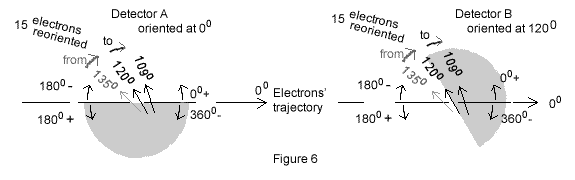In the following description 360 pairs are considered to be emitted; the first pair is emitted with a 10 magnetic tilt, the second with a 20 tilt and so on up till the last, which is emitted with a 3600 tilt. Yet on Figure 6 only three pairs oriented at 1350, 1200, and 1090 have been represented.
As already mentioned because the upper and bottom halves are symmetrical the following is treating the top half only.
And as shown in paragraph "2.5 Bell's theorem local interpretation" the grey and white shades, whether the upper or lower half circumferences are concerned, coincide to one third two third distribution.
In accordance with Equation 2 though the 15 electrons emitted within the 1350→1200 North pole range (labelled 15 electrons reoriented), which should be flashing GREEN in both Detectors, are reoriented to the 1200→1090 range; they will still be flashed GREEN by Detector A , but now they will be flashed RED by Detector B.
In the end the 120 electrons making the former 00→1200 range, which were representing two thirds of the 00→1800 range, are now increased to 120 plus 15 electrons that is 135 or three quarters of the same 00→1800 range.
And the 60 electrons making the former 1200→1800 range, which were representing one third of the 00→1800 range, are now reduced to 60 minus 15 electrons that is 45 in number, which is one quarter of the 00→1800 range.
Whether 360 pairs or millions of pairs are considered this reasoning remains valid.

7. Conclusion

This web-page ultimate goal is to refute both uncertainty and non-locality concepts. It is achieved with a local equation, which fits Quantum Mechanics statistical interpretation.
Another goal is to provide as best as possible a physical explanation of the phenomenon as done in section 6 above.

Statistics does not mandate uncertainty
Both Quantum Equation 1 and local Equation 2 are correctly cloning reality.
Equation 1 uncertainty appears nevertheless incompatible with Equation 2, which instead predicts precisely the electrons individual behaviors.
This incompatibility vanishes noting that Equation 1 statistics does not mandate uncertainty; an ascertainment mathematically proved by EPR, which shows that hidden variables are acting behind Quantum statistics.

Nonlocality is unreasonably commended
When the detectors are set 1200 apart, a pair emitted at 300, as shown Figure 3, is measured GREEN by one detector and RED by the other; all in all the two electrons of a pair maybe measured differently. Yet such measures, when the two colors measured do not comply, were witnessed by human operators even before Equation 2 came into play.
Nonlocality, which states that when one electron is measured the other complies at distance, then cannot refer to the colors measured. Nonlocality must then refer to the fact that the statistics do not coincide to the expected outcome; non locality is a byproduct of unexplained statistics and vanishes with Equation 2.

Bell's inequalities revisited
Based on the wrong one third same colors to two thirds different colors distribution, Bell's famous inequalities are simply disqualified.

EPR's hidden variables reinstated
This interpretation has the definite advantage to confirm that Einstein and colleagues mathematics is right after all. The hidden variable is evidently the particles individual orientation.

References

 Einstein, A., Podolsky, B., Rosen, N.: Can quantum-mechanical description of physical reality be considered complete?
Phys. Rev. 47, 777-780 (1935).
Also available on internet at: EPR
 Bell, J.S.: On the Einstein Podolsky Rosen paradox. Phys. 1, 195-200 (1964)
Available on internet at: Bell's inequalities
[2 a] For everybody explanation: Bell's inequalities for everybody
 Aspect, A., Grangier, P., Roger, G.: Experimental realization of Einstein-Podolsky-Rosen-Bohm Gedanken experiment: a new violation of Bell's inequalities. Phys. Rev. Lett. 49, 91-94 (1982) Available on Internet: Alain Aspect experiments
 American Journal Of Physics; Volume 49; Number 10; Page 940; October 1981.
Bringing home the atomic world: Quantum mysteries for anybody. By N.D.Mermin.
Also available on internet: Mermin's paper

Appendix A: Quantum Mechanics hidden side

This section sheds light on Quantum Mechanics Equation 1 extraordinary alchemy.
As noted in "3. Local Mathematics Conforming to Experiment" the detectors orientations are irrelevant. We can then choose Detector B as being the reference in Equation 1, which can then be rewritten as: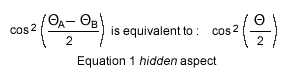Both Equation 1 and its hidden aspect deal with the detectors' orientations rather than with the electrons tilts as does Equation2; the hidden side of Equation 1 suggests that detectors' orientations just as electrons' tilts can be re-oriented in order to describe the entanglement phenomenon. Equation 3, which is inspired by above hidden aspect of Equation 1, does just that:Equation 3 modifies θDetectorNominalOrientation = 1200 into θDetectorEffectiveOrientation = 1350 and θDetectorNominalOrientation = 00 remains unchanged at θDetectorEffectiveOrientation = 00.
In these conditions one quarter to three quarters distribution will be measured provided the emitted electrons are evenly distributed over the full 3600 circumference.
In the end Quantum Mechanics extraordinary alchemy mends nicely with local reality.

Contact:   henriJMsalles[at]gmail.com
Copyright © by Henri Salles. You have the permission to reproduce, print, distribute and post the contents of this webpage, provided you mention proper citation and acknowledgment: www.bell-s-inequalities-revisited.com
or the link: http://bell-s-inequalities-revisited.com by Henri Salles